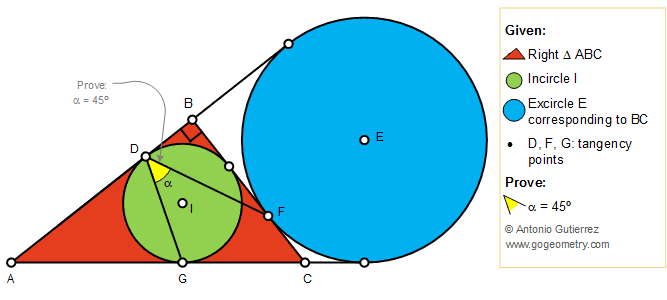Geometry Problem 1412: Right Triangle, Incircle, Excircle, Tangency Points, 45 Degree Angle

Proposition

The figure below shows a right triangle ABC (angle B = 90 degree) with the incircle I tangent to AB and AC at D and G, respectively. The excircle E corresponding to BC is tangent to BC at F. Prove that the measure of the angle FDG is 45 degree.Poster of Problem 1412: Sketching, iPad, Typography, Art